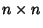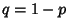## s-Cluster

N.B. A detailed on-line essay by S. Finch was the starting point for this entry.

Let anMatrix have entries which are either 1 (with probability) or 0 (with probability). An-cluster is an isolated group ofadjacent (i.e., horizontally or vertically connected) 1s. Letbe the total number of Site'' clusters. Then the value(1)

called the Mean Cluster Count Per Site or Mean Cluster Density, exists. Numerically, it is found that(Ziff et al. 1997).

Considering instead Bond'' clusters (where numbers are assigned to the edges of a grid) and lettingbe the total number of bond clusters, then(2)

exists. The analytic value is known for,(3)

(Ziff et al. 1997).

References

Finch, S. Favorite Mathematical Constants.'' http://www.mathsoft.com/asolve/constant/rndprc/rndprc.html

Temperley, H. N. V. and Lieb, E. H. Relations Between the Percolation' and Colouring' Problem and Other Graph-Theoretical Problems Associated with Regular Planar Lattices; Some Exact Results for the Percolation' Problem.'' Proc. Roy. Soc. London A 322, 251-280, 1971.

Ziff, R.; Finch, S.; and Adamchik, V. `Universality of Finite-Sized Corrections to the Number of Percolation Clusters.'' Phys. Rev. Let. To appear, 1998.